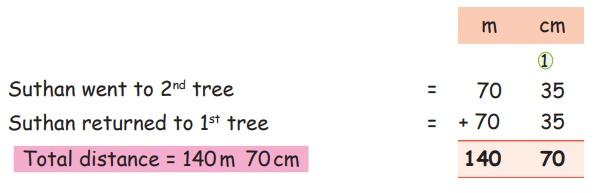Home | | Maths 4th Std | Solving problem involving length and distances

# Solving problem involving length and distances

Solving problem involving length and distances.

Solving problem involving length and distances.

EXAMPLEThe distance between two coconut trees is 70m 35cm.

Suthan walked from first tree to second tree.

Then he returned to first tree. How much distance did he cover?Total distance = 140m 70cm

Activity

Measure the height of any ten of your classmates and write in centimetre.

Group Activity

To go from cuddalore to Chennai, which is the longest route? Find out the distance of the same. Which is the shortest route? Find out the shortest distance.Similarly find the shortest distance between Madurai to Chennai, Trichy to Coimbatore and Chennai to Coimbatore.

Madurai − Dindigul − Trichy − Perambalur − Cuddalore Villupuram − Kanchipuram − Chennai

Trichy − Karur − Coimbatore

Chennai − Vellore − Dharmapuri − Salem − Erode − Coimbatore

Tags : Measurements | Term 1 Chapter 4 | 4th Maths , 4th Maths : Term 1 Unit 4 : Measurements
Study Material, Lecturing Notes, Assignment, Reference, Wiki description explanation, brief detail
4th Maths : Term 1 Unit 4 : Measurements : Solving problem involving length and distances | Measurements | Term 1 Chapter 4 | 4th Maths